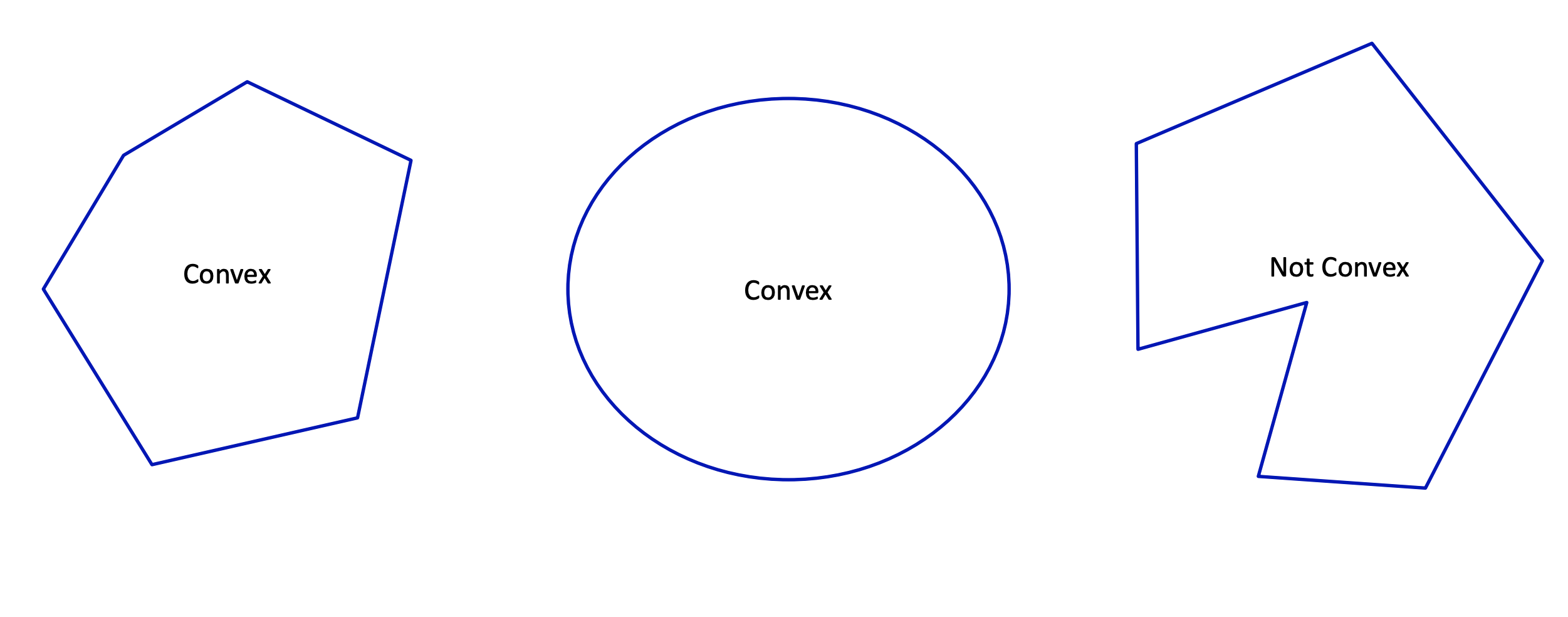A set is convex if line between two points in the set stays in the set.A function is convex if all the points above form a convex set. That is,

• If
• A convex function multiplied by a non-negative constant is convex
• Norms and squared norms are always convex
• The sum of convex functions is convex
• The max of convex functions is a convex function
• The composition of a convex function and linear function , is convex